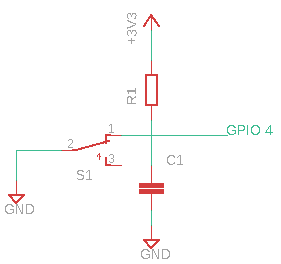# GPIO Input with libgpiod

### 2021-05-10 hardware raspberry-pi

We can use the libgpiod library to configure a GPIO line as input and read it’s value. We’ll create a switch circuit so we can test the value is a logic high or low. More info on libgpiod can be found in my libgpiod intro for installation and usage.

Here is a previous post to blink an LED using libgpiod.

# Input Circuit

Into our Raspberry Pi we’ll use GPIO 4 and configure this pin as an input. We’re going to add some resistors to control the current into this circuit. When the switch is in one direction you can see current will essentially not flow.With some simple math let’s just arbirarily set $I = 1\mu A$ so we can solve for the value of $R_1$.

$V = IR_1$

$R_1 = \frac{V}{I} = \frac{3.3V}{1\mu A} = 3.3M\Omega$

For the value of $C_1$ just chose an arbitary value to debounce the circuit. I’m using a standard $104$ capictor with a value of $100 nF$.

# Using the libgpiod tool gpioget

We can check GPIO Input Line 4 using the gpioget tool:

$gpioget gpiochip0 4 # with the switch off 0 $ gpioget gpiochip0 4 # with the switch on
1

# Using C Code

// file input.c
#include <gpiod.h>
#include <error.h>
#include <stdlib.h>
#include <stdio.h>
#include <string.h>
#include <unistd.h>

struct gpiod_chip *chip;
struct gpiod_line_request_config config;
struct gpiod_line_bulk lines;

int
main(int argc, char *argv[])
{
unsigned int offsets;

int values;
int err;

chip = gpiod_chip_open("/dev/gpiochip0");
if(!chip)
{
perror("gpiod_chip_open");
goto cleanup;
}

// use pin 4 as input
offsets = 4;
values = -1;

err = gpiod_chip_get_lines(chip, offsets, 1, &lines);
if(err)
{
perror("gpiod_chip_get_lines");
goto cleanup;
}

memset(&config, 0, sizeof(config));
config.consumer = "input example";
config.request_type = GPIOD_LINE_REQUEST_DIRECTION_INPUT;
config.flags = 0;

err = gpiod_line_request_bulk(&lines, &config, values);
if(err)
{
perror("gpiod_line_request_bulk");
goto cleanup;
}

err = gpiod_line_get_value_bulk(&lines, values);
if(err)
{
perror("gpiod_line_get_value_bulk");
goto cleanup;
}

printf("value of gpio line %d=%d\n", offsets, values);

cleanup:
gpiod_line_release_bulk(&lines);
gpiod_chip_close(chip);

return EXIT_SUCCESS;
}

# Building and Running

You can build and run with:

$gcc -l gpiod -o input input.c$ ./input